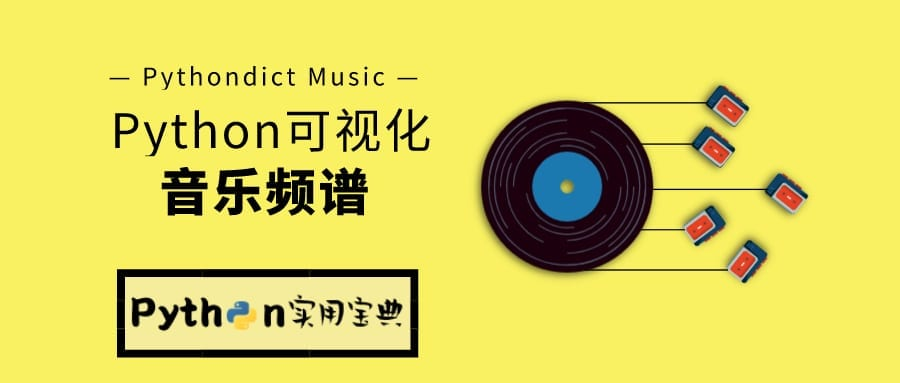# Python 提取音乐频谱并可视化

Python 剪辑音乐就是这么简单

## 1.准备工作

Windows环境下打开Cmd(开始—运行—CMD)，苹果系统环境下请打开Terminal(command+空格输入Terminal)，准备开始输入命令安装依赖。

```pip install pydub
pip install librosa```

## 2.频谱展示

```import matplotlib.pyplot as plt
import librosa.display

# 音乐文件载入
audio_path = 'Fenn.mp3'

# 宽高比为14:5的图
plt.figure(figsize=(14, 5))
librosa.display.waveplot(music, sr=sr)

# 显示图
plt.show()```

```import matplotlib.pyplot as plt
import librosa.display
import numpy as np
from pydub import AudioSegment

# 1秒=1000毫秒
SECOND = 1000
# 音乐文件
AUDIO_PATH = 'Fenn.mp3'

def split_music(begin, end, filepath):
# 导入音乐
song = AudioSegment.from_mp3(filepath)

# 取begin秒到end秒间的片段
song = song[begin*SECOND: end*SECOND]

# 存储为临时文件做备份
temp_path = 'backup/'+filepath
song.export(temp_path)

return temp_path

music, sr = librosa.load(split_music(0, 1, AUDIO_PATH))

# 宽高比为14:5的图
plt.figure(figsize=(14, 5))
librosa.display.waveplot(music, sr=sr)
plt.show() ```

```# 放大
n0 = 9000
n1 = 10000

music = np.array([mic for mic in music if mic > 0])
plt.figure(figsize=(14, 5))
plt.plot(music[n0:n1])
plt.grid()

# 显示图
plt.show() ```

Python 批量下载网易云音乐歌单

Python 制作音乐高潮副歌提取器

Python Django快速开发音乐高潮提取网(1)

Python Django快速开发音乐高潮提取网(2)

Python Django快速开发音乐高潮提取网(3)

Python 超方便超快速剪辑音乐

Python 提取音乐频谱并可视化

¥1¥5¥10¥20¥50¥100¥200 自定义

​Python实用宝典 ( pythondict.com )